•5星
31KB Zzzyzhang 2021-04-16 11:11:12
•5星
401KB m0_52957036 2020-12-16 06:20:16
• 双链表——双链表的定义及其基本操作（初始化、头插法尾插法建表、插入、查找、删除、判空等） 指针 链表 数据结构 算法

文章目录双链表的定义双链表上的操作初始化插入操作建立双链表头插法建立双链表尾插法建立双链表遍历操作求双链表的长度查找操作按值查找按位查找删除操作判空操作完整代码及实例总结 双链表的定义  为什么引入...

双链表的定义

为什么引入双链表？

单链表的结点中只有一个指向其后继的指针，使得单链表要访问某个结点的前驱结点时，只能从头开始遍历，访问后驱结点的复杂度为O(1)，访问前驱结点的复杂度为O(n)。为了克服上述缺点，引入了双链表。

双链表的结点中有两个指针prior和next，分别指向前驱结点和后继结点。

双链表中结点类型的描述：

typedef struct DNode{
int data;  //数据域
struct DNode *prior,*next;  //前驱和后继指针

双链表上的操作

初始化

双链表与单链表一样，为了操作方便也可以加入头结点，那么初始化双链表其实就是定义一个头结点，然后将指针域置空。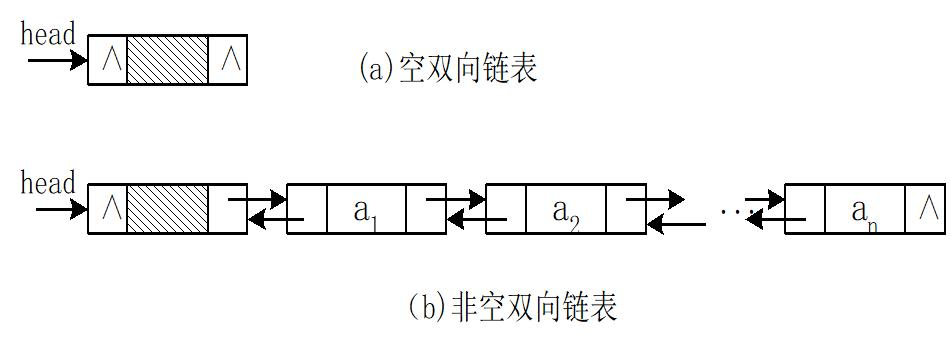//初始化
L->prior = NULL;
L->next = NULL;
}

插入操作

在双链表中p所指的结点之后插入结点*s，其指针的变化过程如下图所示：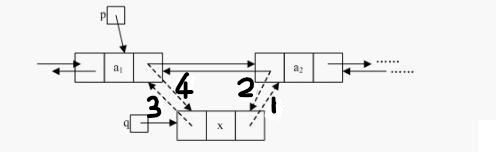实现代码：

//将x插入到双链表L中*p结点的下一个结点
void Insert(DLinkList &L, DNode *p, int x){
DNode *s = (DNode *)malloc(sizeof(DNode));
s->data = x;
s->next = p->next;  //1
p->next->prior = s; //2
s->prior = p;  //3
p->next = s; //4
}

当然，插入操作的语句并不只有这一种顺序，但必须保证1和2两步必须在4步之前，否则*p的后继结点的指针就会丢掉，导致插入失败。

建立双链表

头插法建立双链表

每次在头结点的后面插入新结点。

实现代码：

//头插法建立双链表
InitList(L); //初始化
int x;
cin>>x;
while(x!=9999){
DNode *s = (DNode *)malloc(sizeof(DNode));
s->data = x;
if(L->next == NULL){
s->next = NULL;
s->prior = L;
L->next = s;
}else{
s->next = L->next;
L->next->prior = s;
s->prior = L;
L->next = s;
}
cin>>x;
}
return L;
}

尾插法建立双链表

每次在双链表的尾部插入新结点，为此，应该声明一个尾指针r并始终指向尾结点。

实现代码：

//尾插法建立双链表
InitList(L);
DNode *s,*r=L;
int x;
cin>>x;
while(x!=9999){
s = (DNode *)malloc(sizeof(DNode));
s->data = x;
s->next = NULL;
s->prior = r;
r->next = s;
r = s;
cin>>x;
}
return L;
}

遍历操作

从单链表的第一个结点开始，依次遍历输出结点的数据直到最后一个结点。

实现代码：

//遍历操作
DNode *p = L->next;
while(p){
cout<<p->data<<" ";
p = p->next;
}
cout<<endl;
}

求双链表的长度

从双链表的第一个结点开始，依次遍历每个结点，并计数，直到最后一个结点为止。

实现代码：

//求双链表的长度
DNode *p = L->next;
int len = 0;
while(p){
len++;
p = p->next;
}
return len;
}

查找操作

按值查找

查找值x在L中的位置。与单链表的按值查找是一样的，不会用到前驱结点指针prior。

实现代码：

//按值查找：查找x在L中的位置
DNode *p = L->next;
while(p && p->data != x){
p = p->next;
}
return p;
}

按位查找

查找在双链表L中第 i 个位置的结点。与单链表的按位查找是一样的，不会用到前驱结点指针prior。

实现代码：

//按位查找：查找在双链表L中第i个位置的结点
int j=1;
DNode *p = L->next;
if(i==0)return L;
if(i<1)return NULL;
while(p && j<i){
p = p->next;
j++;
}
return p; //如果i大于表长，p=NULL,直接返回p即可
}

删除操作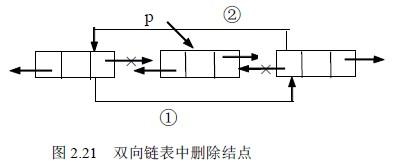将双链表中的第 i 个结点删除。

实现代码：

//删除操作:将双链表中的第i个结点删除
if(i<1 || i>Length(L)){
cout<<"delete failed: index is wrong."<<endl;
return;
}
DNode *p = GetElem(L,i-1);
DNode *q = p->next;
p->next = q->next; //1
q->next->prior = p; //2
free(q);
}

判空操作

判断双链表是否为空。

实现代码：

//判空操作
if(L->next == NULL){
cout<<"L is null"<<endl;
return true;
}else{
cout<<"L is not null"<<endl;
return false;
}
}

完整代码及实例

完整代码：

#include<bits/stdc++.h>
using namespace std;

typedef struct DNode{
int data;
struct DNode *prior,*next;

//初始化
L->prior = NULL;
L->next = NULL;
}

//遍历操作
DNode *p = L->next;
while(p){
cout<<p->data<<" ";
p = p->next;
}
cout<<endl;
}

//求双链表的长度
DNode *p = L->next;
int len = 0;
while(p){
len++;
p = p->next;
}
return len;
}

//头插法建立双链表
InitList(L); //初始化
int x;
cin>>x;
while(x!=9999){
DNode *s = (DNode *)malloc(sizeof(DNode));
s->data = x;
if(L->next == NULL){
s->next = NULL;
s->prior = L;
L->next = s;
}else{
s->next = L->next;
L->next->prior = s;
s->prior = L;
L->next = s;
}
cin>>x;
}
return L;
}

//尾插法建立双链表
InitList(L);
DNode *s,*r=L;
int x;
cin>>x;
while(x!=9999){
s = (DNode *)malloc(sizeof(DNode));
s->data = x;
s->next = NULL;
s->prior = r;
r->next = s;
r = s;
cin>>x;
}
return L;
}

//按值查找：查找x在L中的位置
DNode *p = L->next;
while(p && p->data != x){
p = p->next;
}
return p;
}

//按位查找：查找在双链表L中第i个位置的结点
int j=1;
DNode *p = L->next;
if(i==0)return L;
if(i<1)return NULL;
while(p && j<i){
p = p->next;
j++;
}
return p; //如果i大于表长，p=NULL,直接返回p即可
}

//将x插入到双链表L中*p结点的下一个结点
void Insert(DLinkList &L, DNode *p, int x){
DNode *s = (DNode *)malloc(sizeof(DNode));
s->data = x;
s->next = p->next;
p->next->prior = s;
s->prior = p;
p->next = s;
}

//删除操作:将双链表中的第i个结点删除
if(i<1 || i>Length(L)){
cout<<"delete failed: index is wrong."<<endl;
return;
}
DNode *p = GetElem(L,i-1);
DNode *q = p->next;
p->next = q->next;
q->next->prior = p;
free(q);
}

//判空操作
if(L->next == NULL){
cout<<"L is null"<<endl;
return true;
}else{
cout<<"L is not null"<<endl;
return false;
}
}

int main(){
//尾插法建立双链表，并遍历单链表
cout<<"L: ";
PrintList(L);

DNode *p;
//按值查找
p = LocateElem(L,2);
cout<<"值为2的结点的下一个结点值是："<<p->next->data<<endl;
cout<<"值为2的结点的上一个结点值是："<<p->prior->data<<endl;
//按位查找
p = GetElem(L,3);
cout<<"第三个结点值是："<<p->data<<endl;

//插入操作
Insert(L,p,7);
cout<<"在第三个结点后面插入值为7的结点后L： ";
PrintList(L);

//删除操作
Delete(L, 5);
cout<<"删除第五个结点后L： ";
PrintList(L);

//求表长
cout<<"表长为："<<Length(L)<<endl;;

//判空
Empty(L);
return 0;
}

运行结果：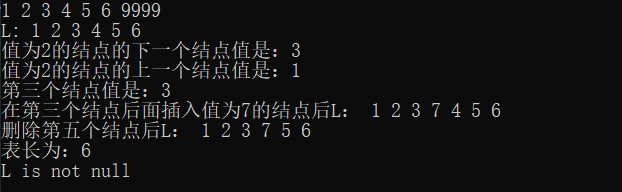总结

双链表与单链表在某些操作上几乎是相同的，比如按位查找，按值查找，求表长，打印链表等等，但由于双链表有指向前驱结点的指针，故双链表在初始化，建立双链表，插入操作，删除操作等函数内需要对前驱结点的指针进行修改。本文中插入和删除操作都是对结点*q的后面进行插入和删除，由于双链表有指向前驱结点的指针，故这些操作也可以转换为在某个结点前的插入删除操作等等。

展开全文weixin_44162361 2021-04-18 10:11:16
• 创建双向链表的算法——C语言实现 数据结构与算法 C语言 双向链表

创建双向链表的算法——C语言实现双向链表也叫双链表，是链表的一种，它的每个节点包含两个指针，分别指向直接后继和直接前驱（头节点的前驱指空，尾节点的后继指空）。所以，从双向链表中的任意一个非前驱非后继...

创建双向链表的算法——C语言实现

双向链表也叫双链表，是链表的一种，它的每个节点包含两个指针，分别指向直接后继和直接前驱（头节点的前驱指空，尾节点的后继指空）。所以，从双向链表中的任意一个非前驱非后继节点开始，都能很方便地访问它的前驱和后继节点。

实际上如果熟练掌握了单向链表的基本操作，双向链表的创建只是每次新建好一个节点后挂链的时候多操作一个指针而已。如果你单向链表的创建还不熟悉，可以看这篇文章中的方法：创建单向链表的三种算法

本文给出创建双向链表的C语言代码，然后在注释中对其进行简单分析。

#include<stdio.h>
#include <stdlib.h>

typedef struct Node{
int data;
struct Node *front,*next;	//双向链表每个节点都有两个指针
}ElemSN;

{
int i;
ElemSN *p=NULL,*q=NULL,*h=NULL;

for(i=0;i<num;i++){
p=(ElemSN *)malloc(sizeof(ElemSN));
p->next=NULL;
p->data=data[i];
if(!h){	//处理头节点
h=p;
h->front=NULL;//头节点的前驱为空
}
else{
q->next=p;
p->front=q;
}
q=p;
}
return h;
}

ElemSN *FindTail(ElemSN *h)//找到双向链表的尾节点
{
ElemSN *p=h;
while(p->next!=NULL){
p=p->next;
}
return p;
}

{
ElemSN *p=NULL;
for(p=h;p!=NULL;p=p->next){
printf("%d\t",p->data);
}
printf("\n双向链表顺序输出结束！\n");
}

{
ElemSN *p=NULL;
for(p=t;p!=NULL;p=p->front){
printf("%d\t",p->data);
}
printf("\n双向链表逆序输出结束！\n");
}

void FreeSpace(ElemSN *h)//释放malloc分配的空间
{
int i;
ElemSN *t = h;
while(t != NULL){
t = h;
h = h->next;
free(t);
}
}

int main(void)
{
int data={3,2,5,8,4,7,6,9};
int num=8;

printf("顺序输出链表值：\n");
printf("逆序输出链表值：\n");

return 0;
}

展开全文FelikZhang 2018-04-04 18:07:23
• 在学习了单链表之后，就顺带学习了双链表的操作。 什么是双链表双链表顾名思义，就是链表由单向的链变成了双向链。 使用这种数据结构，我们可以不再拘束于单链表的单向创建于遍历等操作，大大减少了在使用中存在...

双向链表操作

在学习了单链表之后，就顺带学习了双链表的操作。

什么是双链表？

双链表顾名思义，就是链表由单向的链变成了双向链。 使用这种数据结构，我们可以不再拘束于单链表的单向创建于遍历等操作，大大减少了在使用中存在的问题。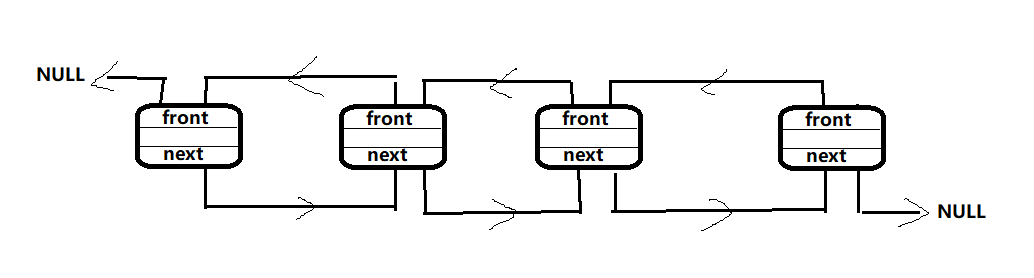在单链表中，我们有一个数据域，还有一个指针域，数据域用来存储相关数据，而指针域则负责链表之间的“联系”。 而在双向链表中，我们需要有两个指针域，一个负责向后连接，一个负责向前连接

/* 单链表的结构 */
struct List{
int data;				//  数据域
struct List *next;			//  指针域
};

/* 双向链表的结构 */
typedef struct List{
int data;			// 	数据域
struct List *next;		//  向后的指针
struct List *front;		//  向前的指针
};

typedef struct List* pElem;
typedef struct List eElem;

同单链表一样，对双向链表的操作也有创建，插入，遍历，删除，销毁

双向链表的创建

在创建双向链表的时候，我们需要初始化的有两个指针。同单链表一样，我们需要一个头指针来标志链表的信息。因此我们可以写出该函数的定义：

pElem CreatList(){
pElem head = (pElem)malloc( sizeof(eElem) );
assert( head != NULL );		//  包含于标准库<assert.h>
}

双向链表的插入

void InsertElem( pElem head , int data ){
printf("The list is empty.\n");		//  头结点为空，则无法插入
return;
}
/*  当双向链表只有一个头结点时 */
pElem addition = (pElem)malloc( sizeof(eElem) );
}
else{
/* 当双向链表不只一个头结点时 */
pElem addition = (pElem)malloc( sizeof(eElem) );
}
}

许多人肯定是被最后四条语句弄得一脸懵逼，都一样。但是当你画出图像时，你就会懂得一切。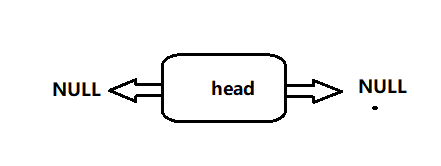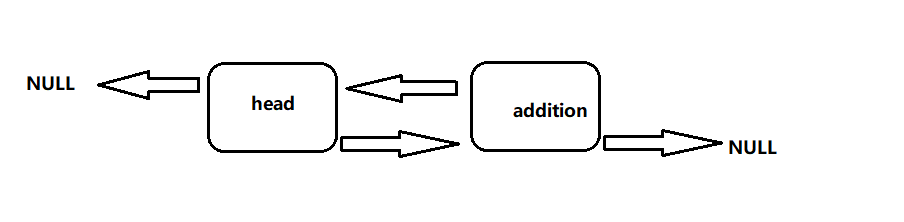具体步骤有以前的博客《创建单链表的头插法与尾插法详解》。

而当链表中不只有一个头结点时，这时有点意思了。

此时，我们要在链表中再插入一个新的结点。 此时，不只要对next 链进行断链，增链，还要对front 链进行同样操作。这样才能完成两条链的连接。

双向链表的遍历

不同于单链表的单向遍历，双向链表提供了操作的便捷性，可前可后的遍历方式简直聊咋咧… 因此在遍历中将实现next 的向后遍历，也会实现front的向前遍历。

printf("-------------------------------\n");
printf("The list is empty.\n");
return;
}
}
}
return;
}

删除一个结点

当删除一个结点的时候，我们要判断在链表中是否存在与之匹配的结点，有的话删除成功，否则失败。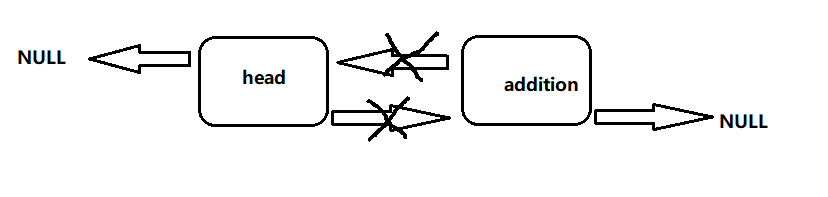void DeleteElem( pElem head , int data ){
printf(""The list is empty.\n);
return;
}
break;
}
}
}

销毁链表

销毁一个双向链表的操作同单链表的相似。指针不断向后运动，每运动一个结点，释放上一个结点。

pElem tmp;
free(tmp);
}
}

这里相对容易理解。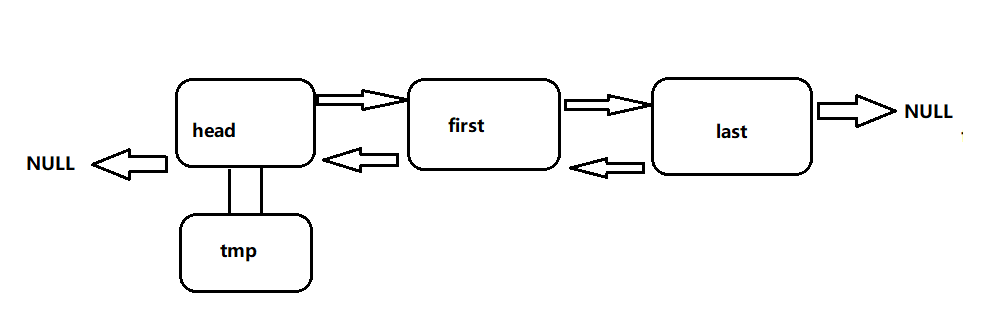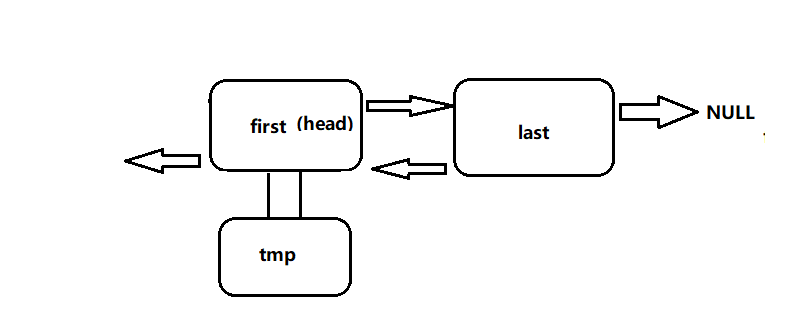上一次执行完之后，头结点已经被释放 ，此时头结点就在第一个数据结点处。 以此往后，最后循环结束，释放最后一个结点。

这样就是双向链表的基本操作

展开全文qq_41028985 2018-11-25 16:18:46
• 双向循环链表的创建及基本操作 数据结构 链表 双向链表 循环链表 c语言

数据结构c语言双向循环链表 双向循环链表在定义上类似于循环单链表，就多了个前指针，以方便于向前查找。在双向链表中需要同时修改两个方向的指针，是单向链表指针的两倍。 完整代码如下： #include <stdio.h>...

数据结构c语言双向循环链表

双向循环链表在定义上类似于循环单链表，就多了个前指针，以方便于向前查找。在双向链表中需要同时修改两个方向的指针，是单向链表指针的两倍。
完整代码如下：

#include <stdio.h>
#include <stdlib.h>
#include <string.h>

typedef struct DuLNode
{
int data;//结点的数据域
struct DuLNode *prior;//结点的后指针域
struct DuLNode *next;//结点的前指针域

DuLNode* InitDuList()//初始化链表  构建一个空的线性表
{
DuLNode* L = (DuLNode*)malloc(sizeof(DuLNode));
if(L==NULL)//判断申请L结点是否为空
{
printf("ERROR!\n");
return 0;
}
L->data = NULL;//定义头结点
L->next = L;
L->prior = L;
}

void Interrupt(void)//创建一个中断函数
{
while(1)//用于检测换行符，使函数脱离scanf的连续输出
if(getchar()=='\n')
break;
}

{
int a,b,i;
DuLNode* p = L;//导入头结点，并创建一个结点s指向头结点
printf("请输入表长： ");
scanf("%d",&a);//输入要插入的链表长度
Interrupt();//中断scanf
printf("请输入表数据： ");
for(i=0;i<a;i++)
{
scanf("%d",&b);//输入要链表数据
DuLNode* s = (DuLNode*)malloc(sizeof(DuLNode));//创建一个结点
s->data = b;
p->next = s;
s->prior = p;
s->next = L;
L->prior = s;
p = p->next;
}
Interrupt();
return 0;
}
{
int a,b,i;
DuLNode* p = L;//导入头结点，并创建一个结点s指向头结点
printf("请输入表长： ");
scanf("%d",&a);//输入要插入的链表长度
Interrupt();
printf("请输入表数据： ");
for(i=0;i<a;i++)
{
scanf("%d",&b);//输入要链表数据
DuLNode* s = (DuLNode*)malloc(sizeof(DuLNode));//创建一个结点
s->data = b;
L->next = s;
s->prior = L;
s->next = p;
p->prior = s;
p = s;
}
Interrupt();
return 0;
}
{
int i=0;
DuLNode* p = L;
while(1)//建立一个死循环，链表最后一个结点指向L(头结点)，以此为判断break循环条件
{
if(p->next == L)
break;
p = p->next;
i++;
}
return i;//返回链表长度
}

{
DuLNode* p = L;//创建一个结点并指向头结点
if(Length(L) == 0)//判断链表是否为空
{
printf("空表\n");
}
else
{
while(1)//建立一个死循环，链表最后一个结点指向L(头结点)，以此为判断break循环条件
{
p = p->next;
printf("%d  ",p->data);
if(p->next == L)//判断break循环条件
break;
}
printf("\n");
}

}

{
DuLNode* p = L;//创建一个结点并指向头结点
int i=0;
if(Length(L)==0)
{
printf("空表无法查询\n");
return 0;
}
else
{
bool a = true;//利用bool类型，来判断链表中是否存在要查找的值
scanf("%d",&e);//要查找的值
Interrupt();
while(1)//建立一个死循环，链表最后一个结点指向L(头结点)，以此为判断break循环条件
{
p = p->next;
i++;
if(p->data == e)
{
printf("第 %d 位  ",i);
a = false;
}
if(p->next == L)
break;
}
if(a)//链表中没有要查找的值
printf("没找到！");
printf("\n");
return 0;
}
}
{
if(Length(L) == 0)//判断链表是否为空
{
printf("空表无法查询！\n");
return 0;
}
scanf("%d",&i);//输入要查找第几位
Interrupt();
int j=0;
DuLNode* p = L;//创建一个结点并指向头结点
if(i<1||i>Length(L))//判断输入的i值是否合理
{
printf("ERROR!\n");
return 0;
}
else
{
for( ;j<i;j++)//利用循环，是p结点指向第i位
p = p->next;
printf("%d\n",p->data);
return 0;
}
}
{
int j;
DuLNode* p = L;//创建一个结点并指向头结点
scanf("%d %d",&i,&e);//输入要插入链表的位置，和值
Interrupt();
if(i<1||i>Length(L)+1)//判断插入链表的位置是否合理
{
printf("ERROR!");
return 0;
}
else
{
DuLNode* s = (DuLNode*)malloc(sizeof(DuLNode));//创建一个新结点
s->data = e;
for(j=1;j<i;j++)//利用循环，是p结点指向第i-1位
p = p->next;
p->next->prior = s;
s->next = p->next;
p->next = s;
s->prior = p;
printf("插入成功\n");
}
}
{
int j;
DuLNode* p = L;//创建一个结点并指向头结点
scanf("%d",&i);//输入要删除链表的结点位置
Interrupt();
if(Length(L)==0)//判断要删除的链表是否为空表
{
printf("ERROR!\n");
return 0;
}
if(i<1||i>Length(L))//判断删除的结点位置是否合理
{
printf("ERROR!\n");
return 0;
}
else
{
for(j=0;j<i;j++)//利用循环，是p结点指向第i位
p = p->next;
p->prior->next = p->next;
p->next->prior = p->prior;
printf("删除成功\n");
return 0;
}
}
int main()
{
int a,b,i=0;
char c;
L = InitDuList();
printf("输入0为尾插法（其他为头插法）：");
scanf("%d",&b);
Interrupt();//中断scanf
if(b==0)
CreatDuList1(L);//尾插法
else
CreatDuList2(L);//头插法
TraverseDuLNode(L);
printf("操作输入序号选择：\n 1：遍历链表\n 2:链表的按位查找\n 3:删除链表元素\n 4:插入链表元素\n 5:链表的按值查找\n 6：表长\n输入#退出\n");
while(1)
{
int f = 0;
printf("请选择：");
scanf("%c",&c);
Interrupt();//中断scanf
switch(c)
{
case '1': printf("遍历并输出链表： ");TraverseDuLNode(L); break;
case '2': printf("链表的按位查找（取值位置）： ");GetElem(L,a); break;
case '3': printf("删除并返回要删除的元素（删除的位置）： ");DuListDelete(L,a); break;
case '4': printf("插入元素（位置 插入的元素）： ");DuListInsert(L,a,a); break;
case '5': printf("链表的按值查找（输入要查找的元素）： ");LocateElem(L,a); break;
case '6': a = Length(L);printf("链表长为： %d\n",a); break;
case '#': f = 1; break;
default: printf("ERROR\n");
}
if (f == 1)
{
printf("已正常退出!\n");
break;
}
}
free(L);
return 0;
}

（完）

展开全文qq_43402544 2020-10-05 16:38:15
• 双链表的尾插法建立输出 双链表

qq_39993896 2017-11-26 17:44:38
• 双向链表各种操作（尾插法建表、初始化、求表长、查找、删除、插入、取值）17计科班教学用 插入 删除

whg841001 2019-04-04 10:00:27
• 数据结构与算法——双链表题解 数据结构 算法 c++

pyx2466079565 2020-09-30 13:01:18
• 双链表基本操作的java代码实现（双链表的创建【头插法】【尾插法】、查找、插入、删除操作） java 链表 数据结构 双向链表

weixin_38334306 2020-07-10 14:30:39
• jianpanliu 2020-06-22 17:10:47
• 数据结构 第4-2讲 双向链表 数据结构 C++ 算法 双向链表

rainchxy 2017-11-21 16:17:41
• qq_44749630 2020-02-05 00:07:41
• qq_44614524 2019-02-18 22:37:57
• 对存储在带头结点的双向链表中的记录利用双向冒泡排序法对其按升序排序 算法 链表 指针 数据结构 c++

weixin_48842132 2020-12-21 16:20:03
• myRealization 2019-10-21 14:41:49
• 双链表结构 数据结构 双链表

weixin_41629698 2019-12-01 12:40:40
• qq_16763983 2020-07-04 11:19:42
• qq_33287871 2020-09-26 15:03:10
• not_give_up_ 2018-05-01 12:26:40
• weixin_43404836 2020-03-11 15:57:12
• 双向链表 数据结构 链表

qq_38193883 2019-07-29 14:27:27
• lilanfeng1991 2013-01-27 14:49:32
• 20-12-16 单向建表和双向链表 java

just_release 2020-12-16 21:26:41
• qq_44614524 2019-02-19 13:53:29
• qq_38853759 2017-10-12 10:14:48
• 双向链表结构实现线性表 c++ 数据结构 链表

qq_41219157 2020-12-10 20:50:59
• 数据结构3_线性表_双向链表 链表 指针 数据结构 单链表 c语言

qq_41473522 2020-04-09 09:41:59
• lilanfeng1991 2013-01-27 14:56:36
• Ulong_ 2018-05-05 11:39:28...